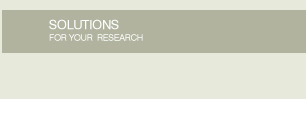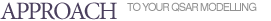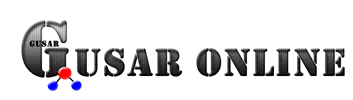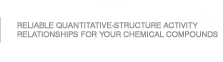GUSAR software was developed to create QSAR/QSPR models on the basis of the appropriate training sets represented as SDfile contained data about chemical structures and endpoint in quantitative terms.
APPLICABILITY DOMAIN
Similarity

Three nearest neighbours from the training set are calculated for each test chemical compound using a similarity value. Similarity of the two chemical compounds is estimated as Pirsons coefficient calculated in the space of independent variables obtained after SCR. The average similarity of three nearest neighbours is used for assessment of the applicability domain (AD) of the model. If the average similarity exceeds the selected threshold (0.7 is default) then the test chemical compound falls in AD of the model and vice-versa. The high value of the threshold was selected the more similar compounds fell in AD of the model.

Leverage

Hat value of leverage was used for domain applicability assessment. Hat values from the leverage matrix representing the "distance"; of the molecule to the model structural space were calculated as:

Leverage = xT(XTX)-1x ,

where x is a vector of descriptors of a query compound, and X is a matrix formed with rows corresponding to the descriptors of molecules from the training set. Hat value of leverage was calculated for each compound of the training set and then a distribution of the obtained values was built.
A warning level of hat value was considered as percentile,  99th is default percentile. Therefore, if a chemical compound from the external test set has hat value exceeded this warning level, then this compound is considered as out of the applicability domain.

Accuracy of three nearest neighbours predictions

For assessment of this type of the applicability domain the follow equation is used:

where ADvalue  the applicability domain value, RMSE3NN  root mean square error of prediction of three most similar compounds from the training set, RMSEtrain  root mean square error of the training set predictions.
Three nearest neighbours from the training set are calculated for each test chemical compound using similarity value. Similarity of the two chemical compounds is estimated as Pirsons coefficient calculated in the space of independent variables obtained after SCR.
If the ADvalue is less than the threshold selected (1 is default) then the test chemical compound falls in AD of the model and vice-versa. Therefore, any chemical compound, whose three nearest neighbours are predicted worse than the whole training set, is considered as out of the applicability domain.